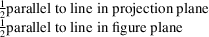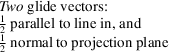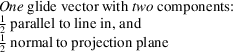International
Tables for
Crystallography
Volume A
Space-group symmetry
Edited by M. I. Aroyo

International Tables for Crystallography (2016). Vol. A, ch. 2.1, p. 146

## Table 2.1.2.2

Th. Hahna and M. I. Aroyoc
 Table 2.1.2.2| top | pdf | Graphical symbols of symmetry planes normal to the plane of projection (three dimensions) and symmetry lines in the plane of the figure (two dimensions)
DescriptionGraphical symbolGlide vector(s) of the defining operation(s) of the glide plane (in units of the shortest lattice translation vectors parallel and normal to the projection plane)Symmetry element represented by the graphical symbolNone mAxial' glide planenormal to projection plane a, b or c
Double' glide planee
Diagonal' glide planen
Diamond' glide plane (pair of planes)parallel to line in projection plane, combined withnormal to projection plane (arrow indicates direction parallel to the projection plane for which the normal component is positive) d
The graphical symbols of the `e'-glide planes are applied to the diagrams of seven orthorhombic A-, C- and F-centred space groups, five tetragonal I-centred space groups, and five cubic F- and I-centred space groups.
Glide planes d occur only in orthorhombic F space groups, in tetragonal I space groups, and in cubic I and F space groups. They always occur in pairs with alternating glide vectors, for instanceand. The second power of a glide reflection d is a centring vector.